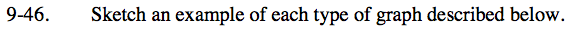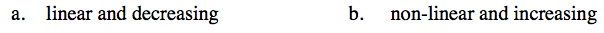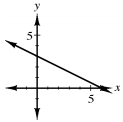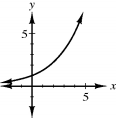Home > ACC7 > Chapter cc39 > Lesson cc39.1.4 > Problem9-46

9-46.
1. Sketch an example of each type of graph described below. Homework Help ✎

1. linear and decreasing

2. non-linear and increasingGraph a line with the formula y = mx + b, where m is negative.

Sketch a line on a graph that goes down as it goes right.

An example of a decreasing linear graph is shown with the equation y = −2x + 6Graph a relationship where either x or y has an exponent greater than 1.

An equation in which x or y has an exponent greater than one will create a curve, rather than a line.

An example of an increasing, non-linear graph is shown with the curve with the equation y3 = x.